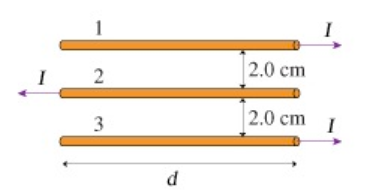# Problem: Assume that in (Figure 1) I = 12A and d = 42cm .What is the magnitude of the force on the second wire?

###### FREE Expert Solution

Magnetic force on a current-carrying conductor:

$\overline{){{\mathbf{F}}}_{{\mathbf{B}}}{\mathbf{=}}{\mathbf{B}}{\mathbf{i}}{\mathbf{L}}}$

Magnetic field around a wire carrying current:

$\overline{){\mathbf{B}}{\mathbf{=}}\frac{{\mathbf{\mu }}_{\mathbf{0}}\mathbf{i}}{\mathbf{2}\mathbf{\pi }\mathbf{r}}}$

Wires carrying current attract each other if the current is flowing in the same direction. The force is repulsive if current flows in opposite directions.

The forces on wire 2 are; F1 repulsive (downward) and F3, repulsive (upward).

97% (472 ratings)###### Problem Details

Assume that in (Figure 1) I = 12A and d = 42cm .

What is the magnitude of the force on the second wire?